MODEL Statement

MODEL dependent = <effects> </ options> ;

The MODEL statement specifies the dependent (response) variable and the independent (regressor) variables or effects. The dependent variable must be numeric. Each term in a MODEL statement, called an effect, is a variable or a combination of variables. You can specify an effect with a variable name or with special notation by using variable names and operators. For more information about how to specify an effect, see the section Specification of Effects in Chapter 42: The GLM Procedure.

Only one MODEL statement is allowed for each PROC SURVEYREG statement. If you specify more than one MODEL statement, the procedure uses the first model and ignores the rest.

Table 94.8 summarizes the options available in the MODEL statement.

Table 94.8: MODEL Statement Options

Option

Description

Produces the ANOVA table

Requests confidence limits

Displays the estimated covariance matrix

Displays design effects

Specifies the denominator degrees of freedom

Displays the inverse or the generalized inverse of thematrix

Omits the intercept

Displays the labels of the parameters

Tunes the estimability checking

Displays parameter estimates

Displays standardized parameter estimates

Specifies whether to use degrees of freedom adjustment

Displays thematrix, or the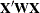matrix

You can specify the following options in the MODEL statement after a slash (/):

requests the procedure compute the adjusted multiple R-square.

ANOVA

requests the ANOVA table be produced in the output. By default, the ANOVA table is not printed in the output.

CLPARM

requests confidence limits for the parameter estimates. The SURVEYREG procedure determines the confidence coefficient by using the ALPHA= option, which by default equals 0.05 and produces 95% confidence bounds. The CLPARM option also requests confidence limits for all the estimable linear functions of regression parameters in the ESTIMATE statements.

Note that when there is a CLASS statement, you need to use the SOLUTION option with the CLPARM option to obtain the parameter estimates and their confidence limits.

COVB

displays the estimated covariance matrix of the estimated regression estimates.

DEFF

displays design effects for the regression coefficient estimates.

DF=value

specifies the denominator degrees of freedom for the F tests and the degrees of freedom for the t tests. For details about the default denominator degrees of freedom, see the section Denominator Degrees of Freedom for details.

I | INVERSE

displays the inverse or the generalized inverse of thematrix. When there is a WEIGHT variable, the procedure displays the inverse or the generalized inverse of thematrix, where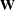is the diagonal matrix constructed from WEIGHT variable values.

NOINT

omits the intercept from the model.

PARMLABEL

displays the labels of the parameters in the Estimated Regression Coefficients table, if the effect contains a single continuous variable that has a label.

SINGULAR=value

tunes the estimability checking. Ifis a vector, define ABS() to be the largest absolute value of the elements of. For a row vectorof the matrix, define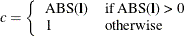If ABS(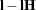) is greater than c*value, then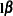is declared nonestimable. Here,
is the matrix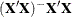. The value must be between 0 and 1; the default is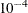.

SOLUTION

displays a solution to the normal equations, which are the parameter estimates. The SOLUTION option is useful only when you use a CLASS statement. If you do not specify a CLASS statement, PROC SURVEYREG displays parameter estimates by default. But if you specify a CLASS statement, PROC SURVEYREG does not display parameter estimates unless you also specify the SOLUTION option.

STB

produces standardized regression coefficients. A standardized regression coefficient is computed by dividing a parameter estimate by the ratio of the sample standard deviation of the dependent variable to the sample standard deviation of the regressor.

specifies whether to use degrees of freedom adjustment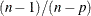in the computation of the matrixfor the variance estimation. If you do not specify the VADJUST= option, by default, PROC SURVEYREG uses the degrees-of-freedom adjustment that is equivalent to the VARADJ=DF option. If you do not want to use this variance adjustment, you can specify the VADJUST=NONE option.
displays thematrix, or thematrix when there is a WEIGHT variable, whereis the diagonal matrix constructed from WEIGHT variable values. The X option also displays the crossproducts vector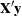or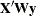.